• ## 样本归一化

千次阅读 2016-05-22 15:44:52
train_data和test_data同时，先归一化 再降维 归一化针对相同维度进行， 注意样本不均衡问题
train_data和test_data同时，先归一化 再降维
归一化针对相同维度进行，
注意样本不均衡问题

归一化：
属于数据preprocess，比方：奇异样本数据(相对于其他输入样本特别大或特别小的样本矢量)  就需要规一化

1.   mapminmax()
将矩阵的每一行归一到[-1 1].
[y,ps] = mapminmax(x1)   %y=(ymax-ymin)*(x-xmin)/(xmax-xmin)+ymin;
其中y是对进行某种规范化后得到的数据，这种规范化的映射记录在结构体ps中。
并不是任何问题都需先对原始data归一化。【原始data规范化后，实际意味着你承认了一个假设：test数据集的每一模式的所有特征分量的max(min)不会大于(小于)train数据集的每一模式的所有特征分量的max(min)，但这条假设显然过于牵强。使用平均值方差法也会有同样类似的问题。】

另一种观点：
当需要对另外一组数据做归一时，比如SVM 中的 training data用以上方法归一，而test data就可以用下面的方法做相同的归一了y2 = mapminmax('apply',x2,PS)当需要把归一的数据还原时，可以用以下命令x1_again = mapminmax('reverse',y1,PS)
Reference:    http://www.matlabsky.com/forum-viewthread-tid-9268-fromuid-18677.html
这个排版更好 http://www.cnblogs.com/avril/archive/2012/09/27/2705050.html

同样还有mapstd   processpca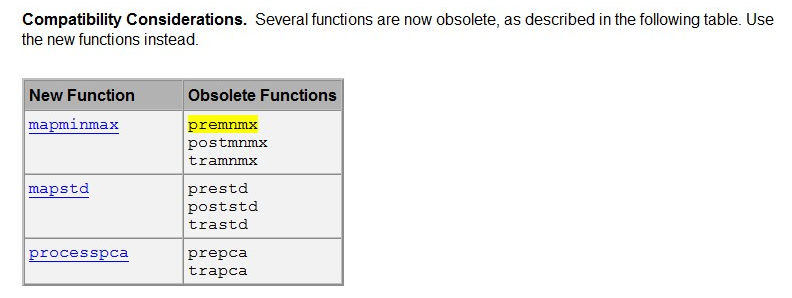注：mapminmax归一到[-1 , 1]；mapstd 归一到单位方差和零均值；一般自己写归一到 (0.1，0.9)
processpca不会用，完全没效果

归一化在 0-1 之间是统计的概率分布，归一化在 -1--+1 之间是统计的坐标分布

train()神经网络的工具箱
归一化是因为sigmoid函数的取值是 0-1 之间，网络最后一个节点的输出也是如此。此处做分类时用[0.9 0.1 0.1]就要比用[1 0 0]要好。


展开全文• 代谢组学中尿液样本归一化的比较研究，王丹丹，李萍，基于尿液样本的代谢组学分析一直是组学研究领域的难点，主要限制因素在于机体摄水量、生理因素以及外界因素都会影响动物的排尿体
• 摘要：由于在训练期间，每一层的输入分布随着前一层参数的改变而发生改变，故训练深度神经网络是复杂的。需求低的学习率与谨慎...批样本归一化允许我们使用更高的学习率，更宽泛的初始化。其表现也类似正则化，在一...
摘要：由于在训练期间，每一层的输入分布随着前一层参数的改变而发生改变，故训练深度神经网络是复杂的。需求低的学习率与谨慎的参数初始化令训练速度变慢，并且训练饱和非线性模型十分困难。我们称这种现象为内部协变量偏移，并且使用归一化层解决这个问题。我们的方法将归一化作为模型架构的一部分，并对每个小批样本执行归一化，这是优势所在。批样本归一化允许我们使用更高的学习率，更宽泛的初始化。其表现也类似正则化，在一定程度上消除了随机失活的使用。应用于最高水准的图像分类模型，批样本达到了相同的准确度，而使用了仅1/14的训练轮数，远远超过了原来的模型。使用一个批样本归一化网络的集合，我们提高了ImageNet分类的最好的公开结果，以4.9%的校验错误（与4.8%的测试错误）达到了前五名，并超越了人类识别准确率。
一、介绍
SGD（stochastic gradient descent）是训练深度网络的有效方法。小批样本尺寸为$m$，估计损失函数的梯度$1/m * ∂l(\bm{x}_{i}, \bm{θ}) / ∂\bm{θ}$计算，有一定优点：小批样本的梯度损失是训练集的梯度损失的估计值，随着批样本尺寸的提升而改善估计质量；由于现代计算平台提供的并行性，在批样本上计算比m次单独计算更加有效。
网络深层的输入受所有前层的影响，故微小的改变会在深层网络放大。因此超参数的初始化需要谨慎的调整。
学习系统的输入分布改变时，会发生协变量偏移，该问题可以用域自适应解决。然而，协变量偏移可以广义的拓宽。考虑$l = F_{2}(F_{1}(\bm{u}, \bm{θ}_{1}), \bm{θ}_{2})$其中$F_{1}$与$F_{2}$是任意变换，参数$\bm{θ}_{1}$与$\bm{θ}_{2}$可以被学习以最小化损失$l$。学习$\bm{θ}_{2}$的过程可以看作$x = F_1(\bm{u}, \bm{θ}_1)$并送入子网络，则$l = F_2(\bm{x}, \bm{θ}_2)$例如一步梯度下降为$\bm{θ}_2 \leftarrow \bm{θ}_2 - a/m \sum_{i=1}^m{∂F_2(\bm{x}_i, \bm{θ}_2)/∂\bm{θ}_2}$其中$m$为批尺寸，$a$为学习率。这与输入为$x$的单独网络$F_2$相同的。因此，输入分布的属性决定了训练的效率，保持$\bm{x}$的分布将十分有利，$\bm{\theta}_2$也不必重新调整来弥补$\bm{x}$在分布上的变化。
保持输入到子网络的分布对于子网络外的层是有益的。考虑S【sigmoid】激活函数$\bm{z} = g(\bm{Wu} + \bm{b})$，其中$\bm{u}$是层的输入，权重矩阵$\bm{W}$与偏置向量$\bm{b}$是层的学习参数，以及$g(x) = 1/(1 + exp\{-x\})$。当$|x|$增加时，$g'(x)$趋于0，这意味着$\bm{x} = \bm{Wu} + \bm{b}$的所有维度，除了有较小的绝对值，否则$\bm{u}$的梯度升降将会消失，模型训练缓慢。然而，由于$\bm{x}$受到$\bm{W}$与$\bm{b}$以及之前所有层参数的影响，在训练时参数的改变很可能将$\bm{x}$的很多维度移动到非线性的饱和区并减缓收敛。该问题随着层数加深而放大。实际上，饱和问题导致的梯度消失经常使用线性整流单元$ReLU(x) = max(x, 0)$，谨慎的初始化以及效地学习率解决。然而，如果能够在网络训练中确保非线性分布输入保持稳定，那么优化便更少的可能陷入饱和区，训练将会加快。
内部协变量偏移【Internal Covariate Shift】指的是深度网络内部节点在训练过程中分布的改变，消除其可以更快的训练。故本文提出了新的原理，称为批样本归一化【Batch Normalization，BN】，使用一个步骤减少内部协变量偏移。其通过一个归一化，固定了层输入的均值与方差。BN也通过减少梯度对参数的规模与初始化的依赖，改善了网络的梯度流。这允许使用更大的学习率，而不必考虑发散的风险。此外，BN正则化了模型并减少了随机失活【Dropout】的必要性。最后，BN通过防止网络陷入饱和模式而使得使用饱和非线性特征成为可能。
二、减少内部协变量偏移
定义内部协变量偏移为由于训练中网络参数改变带来的网络激活值分布的改变。已知如果输入是白的——例如线性变化为0均值与单位方差且不相关，那么网络训练收敛会加快。
考虑通过直接修改网络或根据网络激活值改变优化算法的参数训练，在每个训练步骤中白化激活值。然而，这些修改分布在优化步骤中，梯度下降步骤更新参数的同时需要更新归一化，这减小了梯度的影响。例如，考虑输入为$\bm{u}$的层加入了学习偏置$\bm{b}$，通过在所有训练数据中计算减去激活值的均值归一化其结果，形如$\hat{\bm{x}} = \bm{x} - E[\bm{x}]$，其中$\bm{x} = \bm{u} + \bm{b}$，$X = \{\bm{x}_N\}$是训练集的所有$\bm{x}$值的集合，$E[\bm{x}] = 1/N \sum\bm{x}_i$，若梯度下降忽略$\bm{b}$对$E[\bm{x}]$的依赖，则更新形如$\bm{b} \leftarrow \bm{b} + \Delta\bm{b}$，其中$\Delta\bm{b} \propto - ∂l/∂\hat{\bm{x}}$，故$\bm{u} + \bm{b} + \Delta\bm{b} - E[\bm{u} + \bm{b} + \Delta\bm{b}] = \bm{u} + \bm{b} - E[\bm{u} + \bm{b}]$。因此，将$\bm{b}$的更新与归一化结合以来对层的输出以及损失都没有改变。
上述方法的问题是梯度下降优化没有将归一化考虑在内。接下来确保对于任意参数值，网络总是能产生期望分布的激活值，这会让损失的梯度包含归一化，并依赖于模型的参数$\bm{\theta}$。取层的输入$\bm{x}$，以及训练集的输入集合$X$，归一化可以看作变换$\hat\bm{x} = Norm(\bm{x}, X)$其不仅依赖于训练样本$\bm{x}$，且依赖于所有的样本$X$——如果$\bm{x}$由其他层产生，则它们都还依赖于$\bm{\theta}$。而反馈过程，需要计算雅可比矩阵$∂Norm(\bm{x}, X)/∂\bm{x} \\ ∂Norm(\bm{x}, X)/∂X$如果忽视后一项，则可能导致上述的爆炸。白化输入的代价是昂贵的，因为其需要计算协方差矩阵$Cov[\bm{x}] = E_{\bm{x} \in X}[\bm{x}\bm{x}^T] - E[\bm{x}]E[\bm{x}]^T$以及其平方根倒数，以产生白化激活$Cov[\bm{x}]^{-1/2}(\bm{x} - E[\bm{x}])$。这使得需要寻找另一种不需要分析全部训练集的解决办法来归一化输入。
本文希望通过归一化一个与整个训练数据的统计相关的训练样本的激活值保存网络的信息。
三、通过小批样本统计归一化
因为难以完全白化所有层的输入且并非处处可微，本文做了两个必要的简化。第一，并非共同白化输入与输出的特征，而独立的归一化每一标量特征。对于每层d维输入$\bm{x} = (x_1, ... x_d)$，初始化每个维度$\hat{x}_k = x_k - E[x_k]/(Var[x_k])^{1/2}$其中期望与方差在整个训练集计算。
注意到简化的归一化每层的输入会改变层的表征。归一化s函数的输入会驱使其到达非线性特征的线性区。为了解决这个问题，要确保网络嵌入的变换代表着统一变换。对于每个激活值$x_k$，参数$\gamma、\beta$用于缩放、偏移归一化值$y_k = \gamma_k\hat{x}_k + \beta_k$这些参数使用原始的模型参数单独的学习，并恢复了网络的表征强度。事实上，设定$\gamma_k = Var[x_k]^{1/2}$与$\beta = E[x_k]$能够复原原始的激活值。
在批样本中设置基于整个训练集的每个训练步骤，可以使用整个集合归一化激活值。然而，使用随机优化是不显示的。因此，做出第二个假设：因为使用小批样本随机梯度训练，每个小批样本产生每个激活值的均值与方差估计值。统计用于归一化可以参与梯度反馈。注意到使用小批样本时，计算了每个维度的方差而不是协方差矩阵。在联合情况下，因为小批样本的尺寸小于白化的激活值数量造成了奇异协方差矩阵，便需要正则化。
考虑m尺寸的小批样本$B$，因为归一化独立的应用到每一个激活值，考虑特定的激活值$x$，在一个小批样本中，拥有m个值，形如$B = \{x_1, ..., x_m\}$另其归一化值为$\hat{x}$，线性变换为$y$，则定义变换$BN_{\gamma, \beta} : x \rightarrow y$为批样本归一化变换。描述形如算法1。在算法中，$\epsilon$是被加入小批样本方差的常数以保证数值的有效性。
B = [x_1, ..., x_m]
gamma = 0
beta = 0
# gamma, beta is trainable paramrters
# B is a mini-batch

def BN(x_i, gamma, beta):
mu_B = 1/m * sum(x_i)
# mini_batch mean
sigma2_B = 1/m * sum((x_i - mu_B) ** 2)
# mini-batch variance
hat_x_i = (x_i - mu_B) / (var_B + epsilon) ** (1/2)
# normalize
y_i = gamma * hat_x_i + beta
# scale and shift
return y_i

y = BN(x_i, gamma, beta)

算法1：批归一化变换，应用于整个小批样本的激活值$x$。
BN变换可以添加到网络用于操作激活值。注意到BN变换不独立于每个训练样本的激活过程，BN还依赖于训练样本与小批样本中的其他样本。缩放偏移值$y$传递到其他层，归一化激活值$\hat{x}$在变换的内部，但其存在是至关重要的。只要每个小批样本从相同的分布抽样，并忽视$\epsilon$，任何$\hat{x}$拥有0期望与1方差。
在训练中，需要反馈通过该变换的损失$l$的梯度，使用链式法则，形如$∂l/∂\hat{x_i} = ∂l/∂y_i ·\gamma \\ ∂l/∂\sigma_B^2 = \sum_{i=1}^m ∂l/∂\hat{x_i}·(x_i - \mu_B)·-(\sigma^2_B + \epsilon)^{-3/2}/2 \\ ∂l/∂\mu_B = (\sum_{i=1}^m ∂l/∂\hat{x}_i·-1/(\sigma^2_B + \epsilon)^{1/2} ) + ∂l/∂\sigma_B^2·\sum_{i=1}^m-2(x_i - \mu_B)/m \\ ∂l/∂x_i = ∂l/∂\hat{x_i}·1/(\sigma^2_B + \epsilon)^{1/2} + ∂l/∂\sigma_B^2 · 2(x_i - \mu_B)/m + ∂l/∂\mu_B · 1/m \\ ∂l/∂\gamma = \sum_{i=1}^m ∂l/∂y_i · \hat{x_i} \\ ∂l/∂\beta = \sum_{i=1}^m ∂l/∂y_i$因此，BN变换可微并将归一化响应引入了网络。
3.1 使用批样本归一化网络训练与预测
为了批样本归一化网络，指定一个激活值的子集并将BN变换插入其中，如算法1。之前，层的输入为$\bm{x}$，而现在为$BN(\bm{x})$。使用BN的网络可以使用批梯度下降或随机梯度下降，并使用批样本尺寸$m > 1$，或其他优化方法。依赖于批样本的激活值归一化可以有效的训练，但在预测中并非需要的。一般的希望输出仅依赖于网络。为了达到该目的，网络训练完成后，对比小批样本更大的统计群体使用归一化$\hat\bm{x} = (\bm{x} - E[\bm{x}])/(Var[\bm{x} + \epsilon])^{1/2}$忽略$\epsilon$，归一化激活在训练时有着0均值与1方差。使用无偏方差估计$Var[\bm{x}] = m/(m - 1)·E_B[\sigma^2_B]$，其中期望为m大小尺寸的训练批样本，$\sigma^2_B$是其方差。使用滑动平均，可以追踪模型训练时的准确率。在预测时，均值与方差固定，归一化只是简单的$\gamma, \beta$组成的线性变换。算法2总结了训练批样本归一化网络的步骤。
N = Net(theta)
x = [x_0, ... x_K][k]
# N is the network with trainable paramrters theta
# x is the subset of activations [x_k], k = 1:K

N_BN_train = N
# Training the BN net
for k in range(K):
N_BN_train[l] = BN(x_k)
# Add the y_k = BN(x_k, gamma, beta) to N_BN_train
N_BN_train[l][x_k] = y_k
# Modify each layer in N_Bn_train with x_k to take y_k instead

theta = optimize(theta)
[gamma_0, ..., gamma_k] = optimize([gamma_0, ..., gamma_k])
[beta_0, ... beta_k] = optimize([beta_0, ... beta_k])
# Train N_BN_train to optimize the parameters

N_BN_infer = N_BN_train
# Inference BN network with frozen parameters

for i in range(K):
B = mini_batches
# The size of B is m
E(x_k) = E(mu_B)
Var(x_k) = m/(m - 1) * E(sigma_B^2)
N_BN_[l][y_k = BN(x_k)] = [y_k = gamma / (Var(x_k) + epsilon) ** (1 / 2) * x_k +
(beta - gamma * E(x_k) / (Var(x_k) + epsilon) ** (1 / 2))]
# Replace the transform BN to linear

算法2：训练批样本归一化网络
3.2 批样本归一化卷积网络
批样本归一化可以应用于任何网络的激活值集合。考虑元素级的非线性仿射变换$z = g(Wu + b)$其中$W, b$是模型学习的参数，$g(·)$是非线性函数。使用$z = g(BN(Wu))$来代替，其中$b$由于计算均值而被忽视。
对于卷积层，额外的考虑了卷积属性的归一化——使得相同特征图在不同的位置的元素以相同的方法归一化，在一个小批样本所有位置的所有激活值联合归一。在算法1中，令$B$是特征图所有值的集合，因此对于m尺寸的小批样本与pxq尺寸的特征图，使用尺寸为mxpxq的小批样本。每一个特征图都将学习到参数$\gamma^{(k)}, \beta^{(k)}$，而不是每个激活值。算法2也做相似的修改。
3.3 批样本归一化使用高学习率
传统的深度网络在太高的学习率下会发生梯度弥散或消失，或陷入局部最小值。通过归一化激活值，使得参数的变化不会放大到过大，防止了次优化。对于实例，其放置了非线性过饱和。
BN也使得参数的尺度更有弹性。一般来说，大的学习率增加了参数的规模，会在反向传播时放大梯度，导致模型的爆炸。然而，BN使得反向传播不受参数尺度的影响，例如对于缩放因子$a$，有$BN(Wu) = BN(a(Wu))$而$∂BN(a(Wu)) / ∂u = ∂BN(Wu) / ∂u \\ ∂BN(a(Wu)) / ∂(aW) = 1/a · ∂BN(Wu) / ∂W$缩放并没有影响该层的雅可比矩阵已经相应的梯度传播。此外，更大的权重导致了小梯度，而BN会保证参数的稳定。
3.4 批样本归一化正则化模型
使用BN层训练时，一个训练样本在小批样本中与其他样本联结，而训练网络不再为给出的单独的训练样本产生确定的值。在实验中，可以发现这种影响对于网络的泛化有益。鉴于随机失活【Dropout】典型的应用于防止过拟合，但在BN网络中发现其可以被移除，或减小比例。

四、实验

五、结论
本文提出了一种机制，显著的加速了深度网络的训练。其基于协变量偏移，通过从网络的激活值中移除其以帮助训练。仅仅在高水准的的图像分类模型中加入批样本归一化，就可以显著提高训练的速度。此外，增加学习率并移除随机失活以及其他变动，便打败了之前单独图像分类网络的水准。通过BN训练的多种模型比ImageNet中的知名系统都有显著性提高。


展开全文人工智能 神经网络
• 一般，我们需要对神经网络的输入样本进行归一化，通常有多种选择，比如归一化到[0,1]，或归一化到[-1,1]。 这两种方法，哪种归一化方法更好呢？还是没有区别。 现在的实验经验，一般归一化后的数值沿原点对称最好...
一般，我们需要对神经网络的输入样本进行归一化，通常有多种选择，比如归一化到[0,1]，或归一化到[-1,1]。

这两种方法，哪种归一化方法更好呢？还是没有区别。

现在的实验经验，一般归一化后的数值沿原点对称最好，即归一化到[-1,1]可以期待取得更好的效果。

因此，推荐在归一化时，把样本数据归一化到[-1,1]，或者其他相对于原点对称的范围。

对应的，似乎使用双极性激活函数(tanh)优于单极性激活函数(sigmoid),因为tanh的激活值范围[-1,1]，而sigmoid的激活值范围是[0,1]

展开全文• 机器学习——SVM之python实现数据样本标准化和归一化
目录

一、标准化和归一化的目的

1、标准化

2、归一化

二、标准化和归一化常用的理论公式

1、归一化

2、标准化

三、python实现SVM样本数据标准化和归一化

1、标准化

2、归一化

本文源代码：《机器学习——支持向量机SVM之python实现简单实例一》

一、标准化和归一化的目的

1、标准化（scale）

将每个数据特征数据均值变为0，标准差变为1

标准化的目的是为了下一步数据的处理提供方便，而进行数据缩放等变化

数据的标准化是将数据按比例缩放，使之落入一个小的特定区间。在某些比较和评价的指标处理中经常会用到，去除数据的单位限制，将其转化为无量纲的纯数值，便于不同单位或量级的指标能够进行比较和加权。

目前数据标准化方法有多种，归结起来可以分为直线型方法(如极值法、标准差法)、折线型方法(如三折线法)、曲线型方法(如半正态性分布)。不同的标准化方法，对系统的评价结果会产生不同的影响，然而不幸的是，在数据标准化方法的选择上，还没有通用的法则可以遵循。

2、归一化（normalization）

1 把数变为（0，1）或者（-1，1）之间的小数

归一化的目的是为了消除不同数据之间的量纲，方便数据比较和共同处理
主要是为了数据处理方便提出来的，把数据映射到0～1范围之内处理，更加便捷快速，应该归到数字信号处理范畴之内。
2 把有量纲表达式变为无量纲表达式
归一化是一种简化计算的方式，即将有量纲的表达式，经过变换，化为无量纲的表达式，成为纯量。 比如，复数阻抗可以归一化书写：Z = R + jωL = R(1 + jωL/R) ，复数部分变成了纯数量了，没有量纲。
另外，微波之中也就是电路分析、信号系统、电磁波传输等，有很多运算都可以如此处理，既保证了运算的便捷，又能凸现出物理量的本质含义。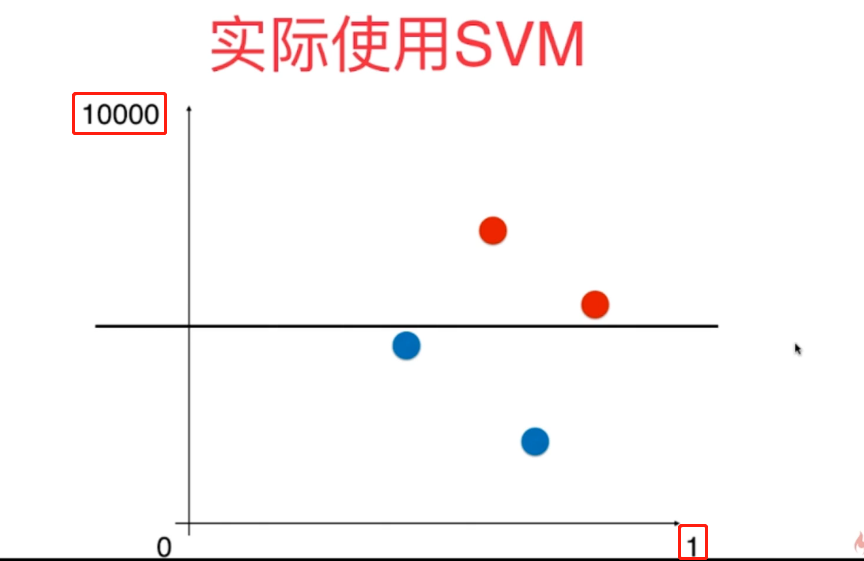实际上是如下图所示的决策边界比较合理，这就需要通过标准化来进行实现，支持向量4个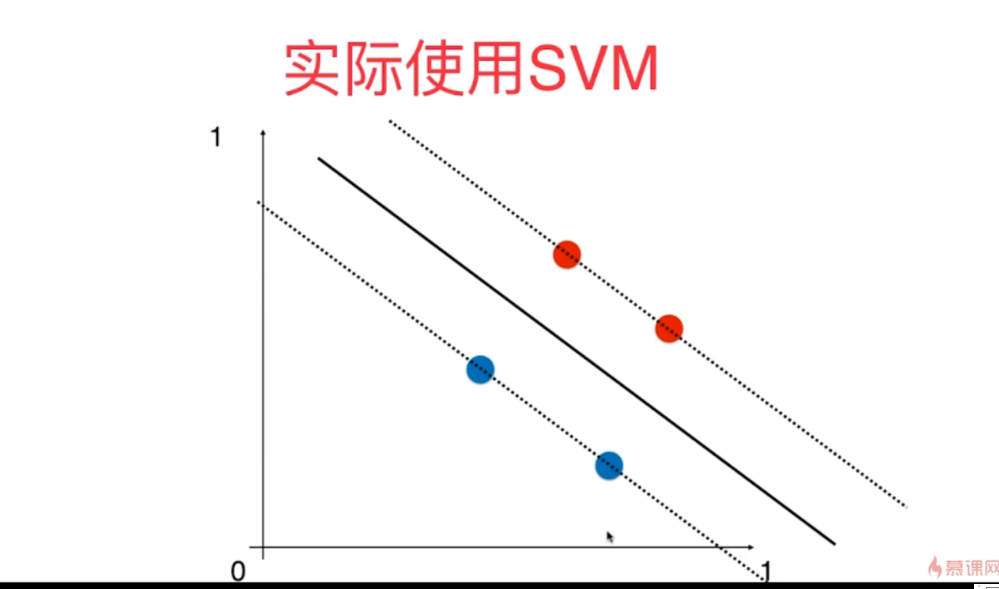二、标准化和归一化常用的理论公式

具体怎么计算一个矩阵的均值和方差网上很多不再赘述

1、归一化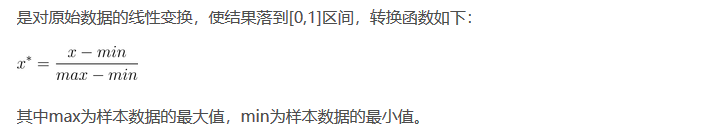2、标准化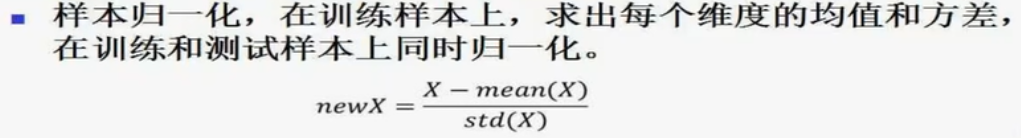三、python实现SVM样本数据标准化和归一化

建议自己按照公式进行编程

1、标准化

标准化的公式很简单，步骤如下

1.求出各变量（指标）的算术平均值（数学期望）xi和标准差si ；
2.进行标准化处理：
zij=（xij－xi）/si
其中：zij为标准化后的变量值；xij为实际变量值。
3.将逆指标前的正负号对调。
标准化后的变量值围绕0上下波动，大于0说明高于平均水平，小于0说明低于平均水平。

常用

from sklearn import preprocessing
import numpy as np
......
x_scaled = preprocessing.scale(x)#x是要进行标准化的样本数据
......

除了用scale函数，还可以用以下几种方法对数据进行标准化

#样本数据归一化，标准化
from sklearn.preprocessing import StandardScaler
standardscaler = StandardScaler()
#对数组x遍历，对每一个样本进行标准化
standardscaler.fit(x)
#返回类StandardScaler()<class'sklearn.preprocessing._data.StandardScaler'>
x_standard = standardscaler.transform(x)#返回标准化后的样本集

def z_score(x, axis):
x = np.array(x).astype(float)
xr = np.rollaxis(x, axis=axis)
xr -= np.mean(x, axis=axis)
xr /= np.std(x, axis=axis)
# print(x)
return x

def standardize(x):
return (x - np.mean(x))/(np.std(x))

2、归一化

def normalize(x):
return (x - np.min(x))/(np.max(x) - np.min(x))


展开全文python 机器学习
• 数据归一化处理是数据挖掘中一项基本的工作，不同评价指标往往具有不同的量纲和量纲单位，这样 的情况会影响到数据分析的结果，为了消除指标之间量纲的影响，需要进行数据标准化处理，以解决 数据指标之间的可比性...
• 数据的标准化（normalization）是将数据按比例缩放，使之落入一个小的...　其中最典型的就是数据的归一化处理，即将数据统一映射到[0,1]区间上，常见的数据归一化的方法有： min-max标准化(Min-max normalization)atan long
• 归一化化定义：我是这样认为的，归一化化就是要把你需要处理的数据经过处理后（通过某种算法）限制在你需要的一定范围内，因为我们得到的样本数据中可能一个特征向量的某几个元素的值非常大，使得特征数据不在一个...
• [train_scale,test_scale,ps] = scaleForSVM(train...ymin：归一化范围下限（可不输入，默认为0） ymax：归一化范围上限（可不输入，默认为1） 输出： train_scale：归一化后的训练集 test_scale：归一化后的测试集 ...机器学习 模式识别
• ## 归一化与反归一化

千次阅读 2020-09-28 10:28:13
归一化的具体作用是归纳统一样本的统计分布性。归一化在0-1之间是统计的概率分布，归一化在-1–+1之间是统计的坐标分布。归一化有同一、统一和合一的意思。无论是为了建模还是为了计算，首先基本度量单位要同一，...
• 机器学习算法中要求样本间的距离就要使用数据归一化，把数据映射到同一尺度。 数据归一化是为了解决量纲的问题，使数据映射到同一尺度。举2个例子：比如两个特征为月收入和和身高。月收入范围5000元-30000元，身高为...机器学习
• 机器学习的一个环节：特征归一化。连续回答下面几个问题，相信也懂个七七八八。 1.什么是特征归一化？ 将特征向量映射到同一分布、缩放到某一个范围内、去量纲的行为，称之为特征归一化。 2.特征归一化的方法有...
• 当算法需要使用梯度下降的方法求解最优解（比如逻辑回归）或者该算法计算样本点距离时（比如KNN）须使用归一化处理。 如何归一化 线性归一化 Min-Max 归一化：比较适用在数值比较集中的情况。实际使用中可以用...标准化 数据挖掘 机器学习
• 1，标准归一化。 　将原始数据集归一化为均值为0、方差1的数据集，归一化公式如下： x∗=x−μδ x^* = \frac{x-\mu}{\delta} 其中μ\mu为所有样本数据的均值，δ\delta为所有样本数据的标准差。 2，最大最小归一...matlab
• 1.5 BN的优势2 样本归一化（IN）3 权重归一化（WN）4 层归一化（LN）5 组归一化（GN）参考 0 归一化（N） 1 批归一化（BN） Batch Normalization 1.1 提出背景 2015，Google，Sergey Ioffe&Christian Szegedy ...深度学习 神经网络 数据分析
• 最大最小值归一化和L2范数归一化是很常用的两种数据预处理方法，本文总结了这两种方法的一些特点。...之所以要按照不同特征维度进行归一化，是因为如果将每个样本进行最大值最小值归一化，数据的分布信息将会丢失。...
• 人脸照片1000多张，基本都是外国人，可用于opencv开发。还有简单的图片归一化程序代码，一起打包，2分你买不了吃亏，2分你买不了上当！
• 数据归一化 文章目录数据归一化一、最值归一化二、均值方差归一化三、...样本间的距离时间所主导，这样在样本1以[1, 200]输入到模型中去的时候，由于200可能会直接忽略到1的存在，因此我们需要将数据进行归一化。比...
• 归一化定义：归一化就是要把需要处理的数据经过处理后（通过某种算法）限制在你需要的... 归一化的具体作用是归纳统一样本的统计分布性。 归一化在0-1之间是统计的概率分布，归一化在某个区间上是统计的坐标分布。 ...
• batch_normalization就是在batch方向上，做样本归一化
• 数据标准化（归一化）处理是数据挖掘的一项基础工作，不同评价指标往往具有不同的量纲和量纲单位，这样的情况会影响到数据分析的结果，为了消除指标之间的量纲影响，需要进行数据标准化处理，以解决数据指标之间的...机器学习 数据挖掘
• 用opencv的traincascade.bat来训练人头，内有人头正样本和负样本，还有归一化尺寸的matlab代码，不需要建立工程，只需简单设置参数就可以进行训练，同时内txt文档还有使用的过程，使训练更简答...